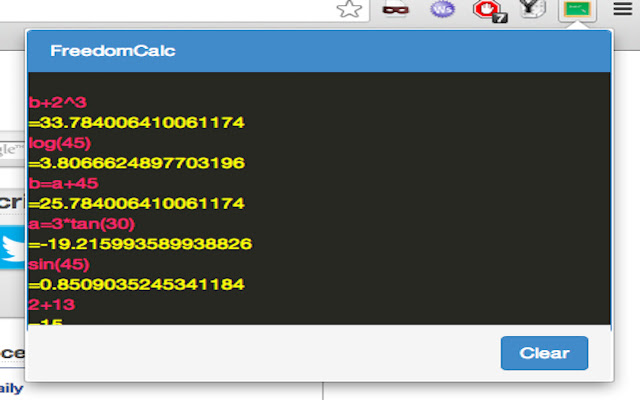# FreedomCalc

CRX id

## pgkcfgmddfnghiihjjlbacjjdpcnkogaDescription from extension meta

## Free text calculator extension, You can perform basic to complex mathamatical calculations without irritating button clicks.

Image from storeDescription from store Free text based basic and scientific calculator, where you can just perform calculation by typing in in free text form like 2*log(30) etc and press enter. You can also perform unit conversions by typing "30mm in inch". You can chain Operations, with variables, like eg. a=3+5 a^10 Where value of "a" from first step will be used to calculate second step, you can use any number of variables across operations. An inbuilt variable "ans" can be used to get answer of previous calculation, eg. 10+12+13+24 ans/4 Second step above use the summation form first step and divided it by 4. You can select any mathematical text in browser while accessing and website, right click and select FreedomCalc to get result of the calculation. All Available operations: x + y (add) x - y (subtract) x * y (multiply) x .* y (element-wise multiply) x / y (divide) x ./ y (element-wise divide) x % y (mod) x ^ y (power) x ^ y (element-wise power) -y (unary minus) y' (transpose) y! (factorial) x = y (assignment) x : y (range) x in y (unit conversion) x == y (equal) x != y (unequal) x < y (smaller) x > y (larger) x <= y (smallereq) x >= y (largereq)

Installs
55 history
Category
Rating